# Printable Math Worksheets

📆 20 May 2022
🔖 Math Category
📂 Gallery Type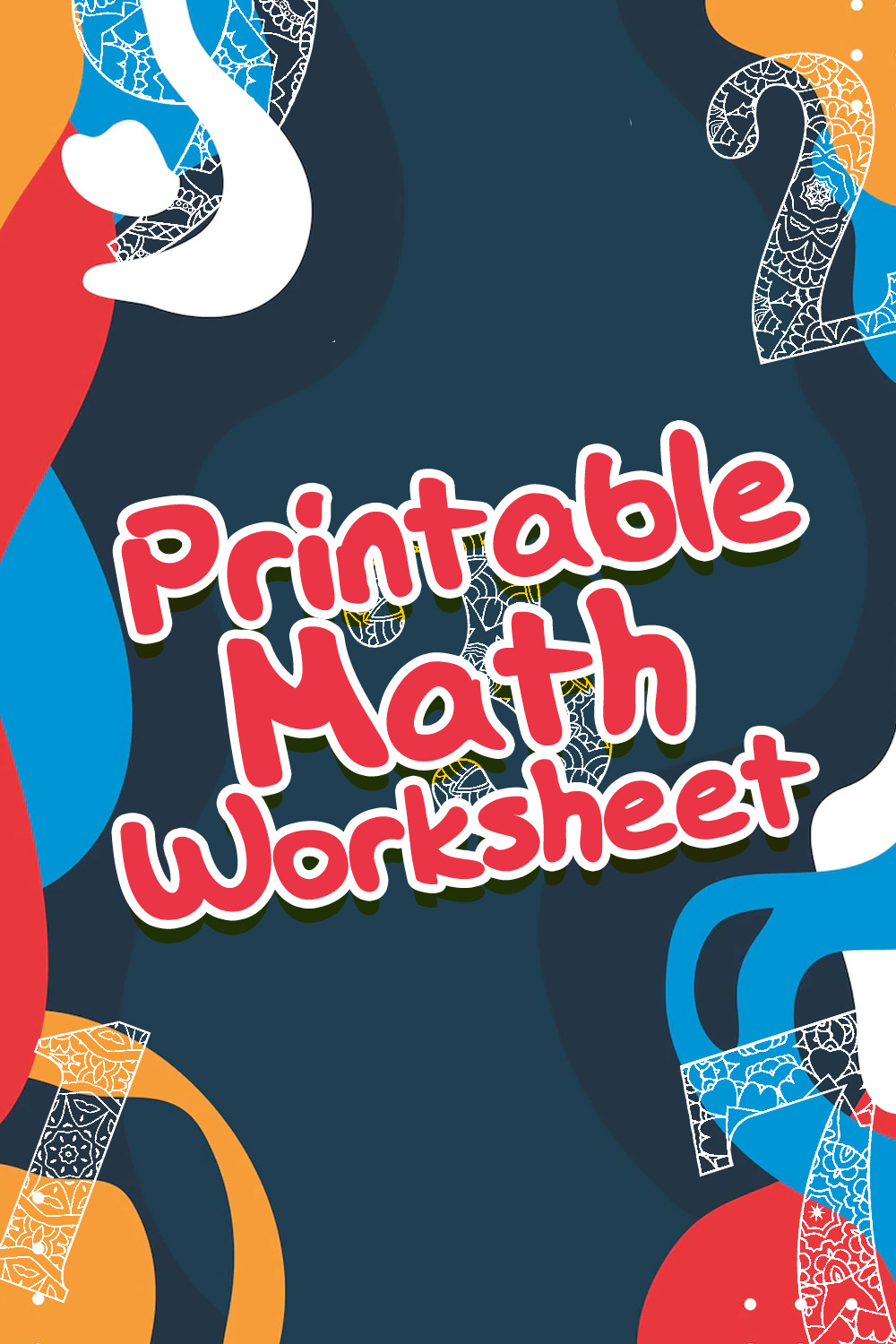10 Images of Printable Math Worksheets

Find 10 best printable math worksheets here to teach your student!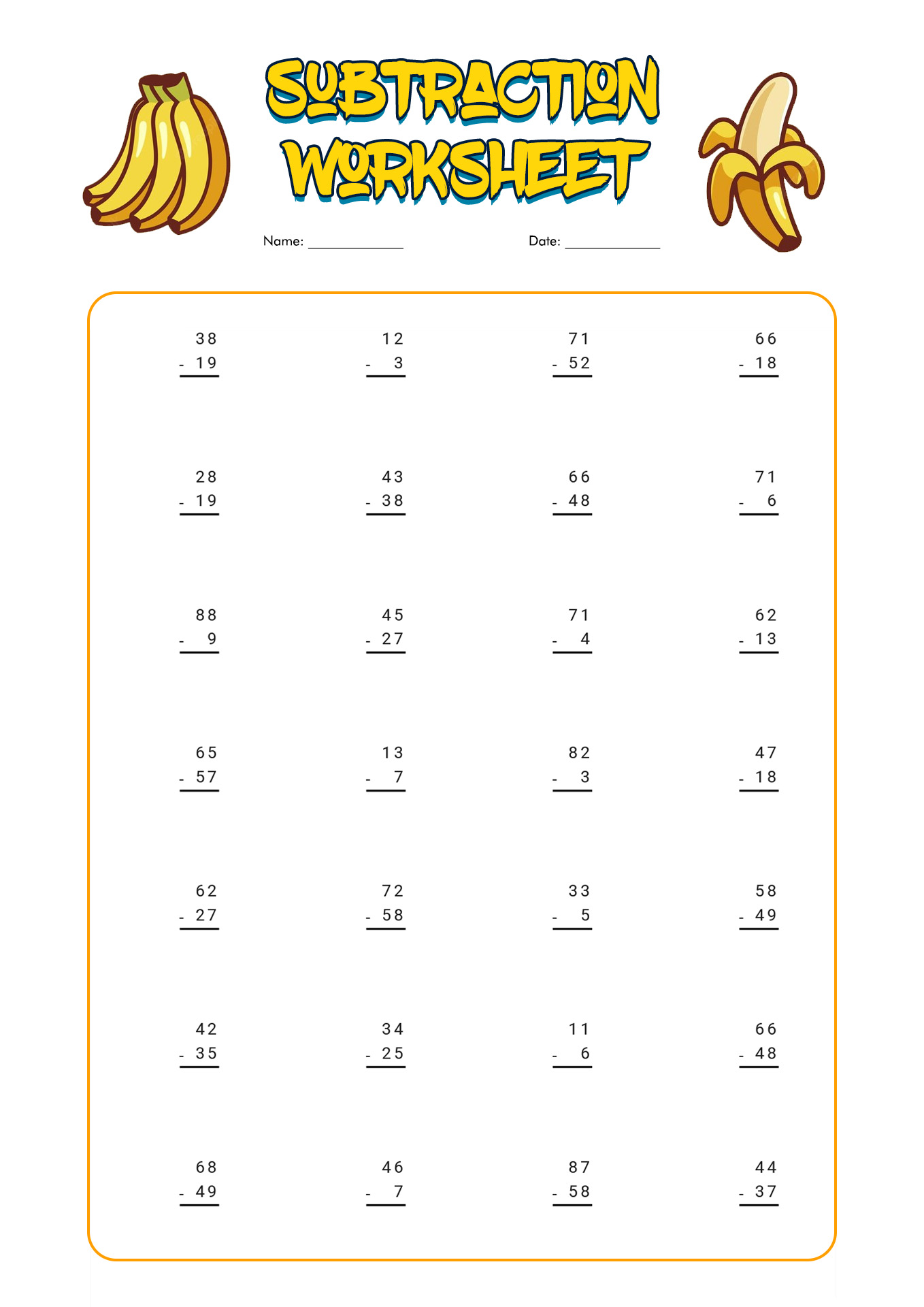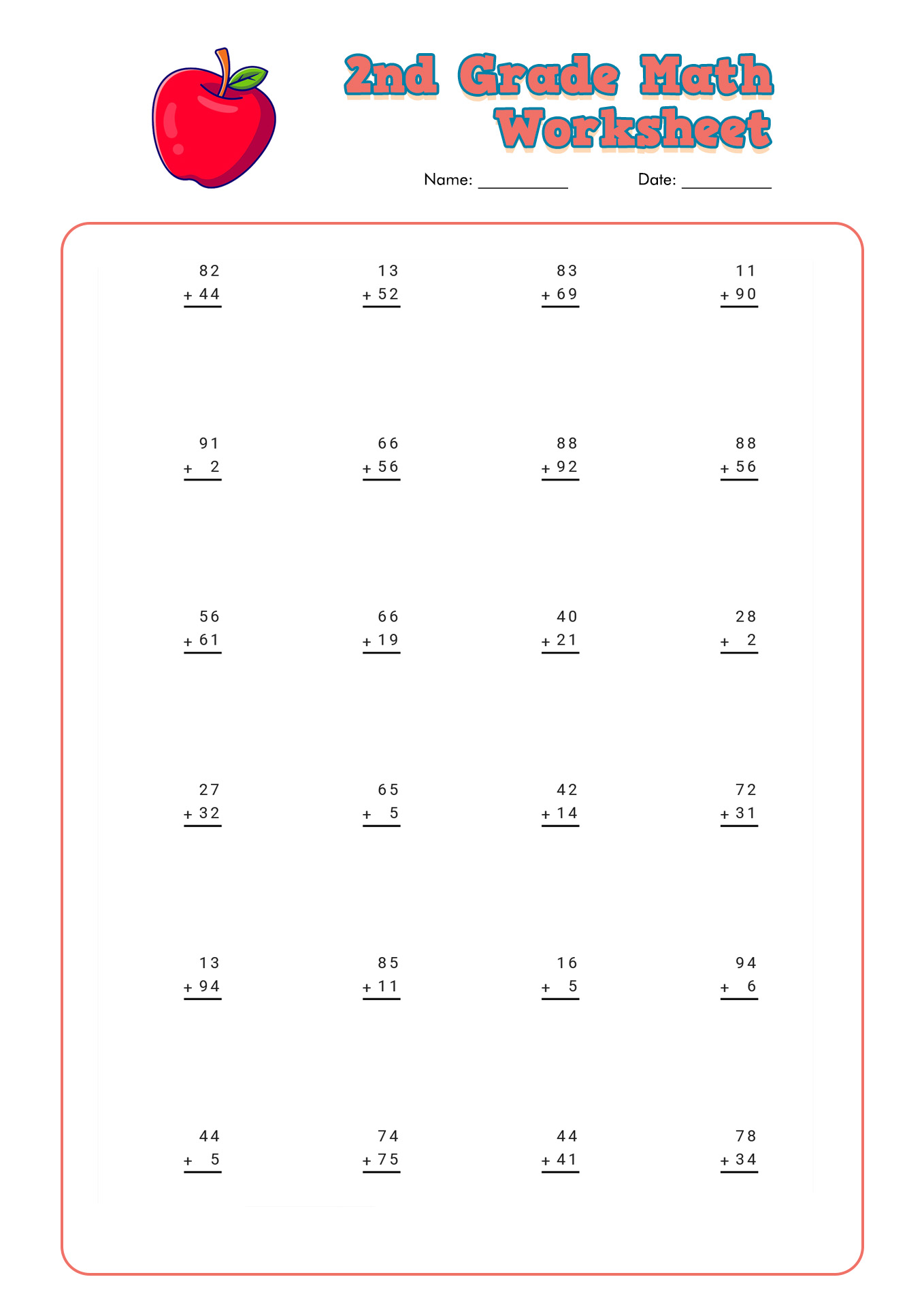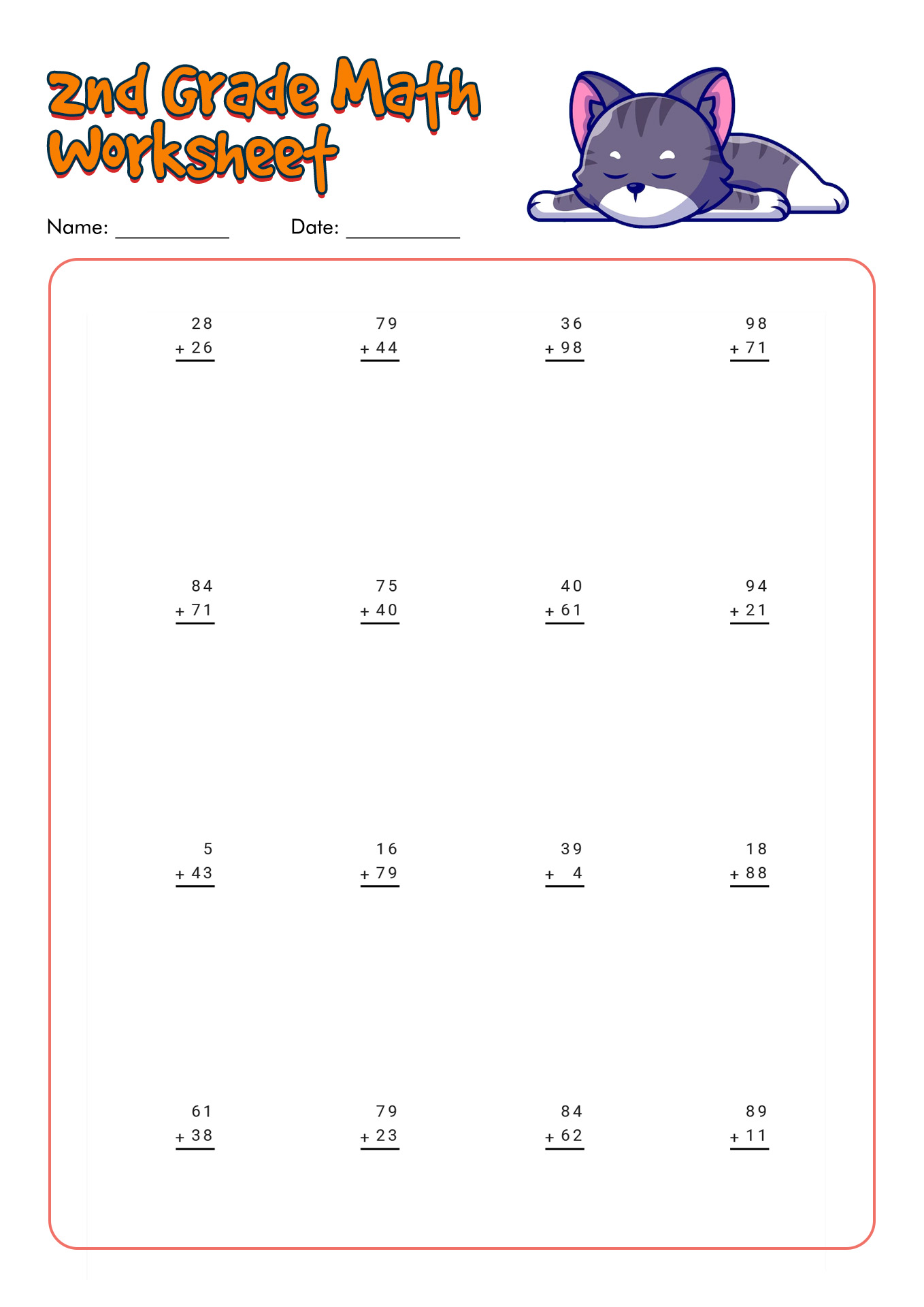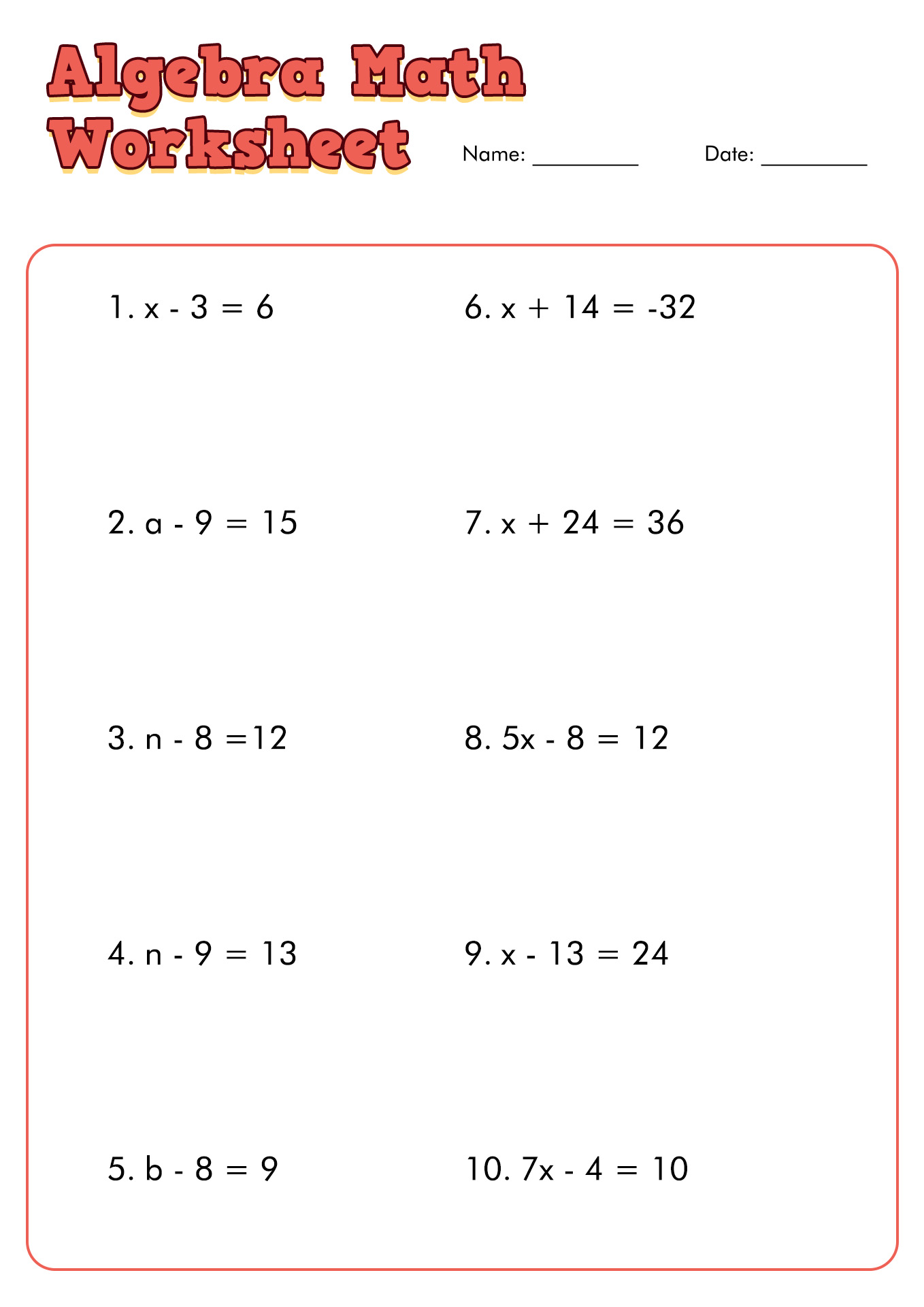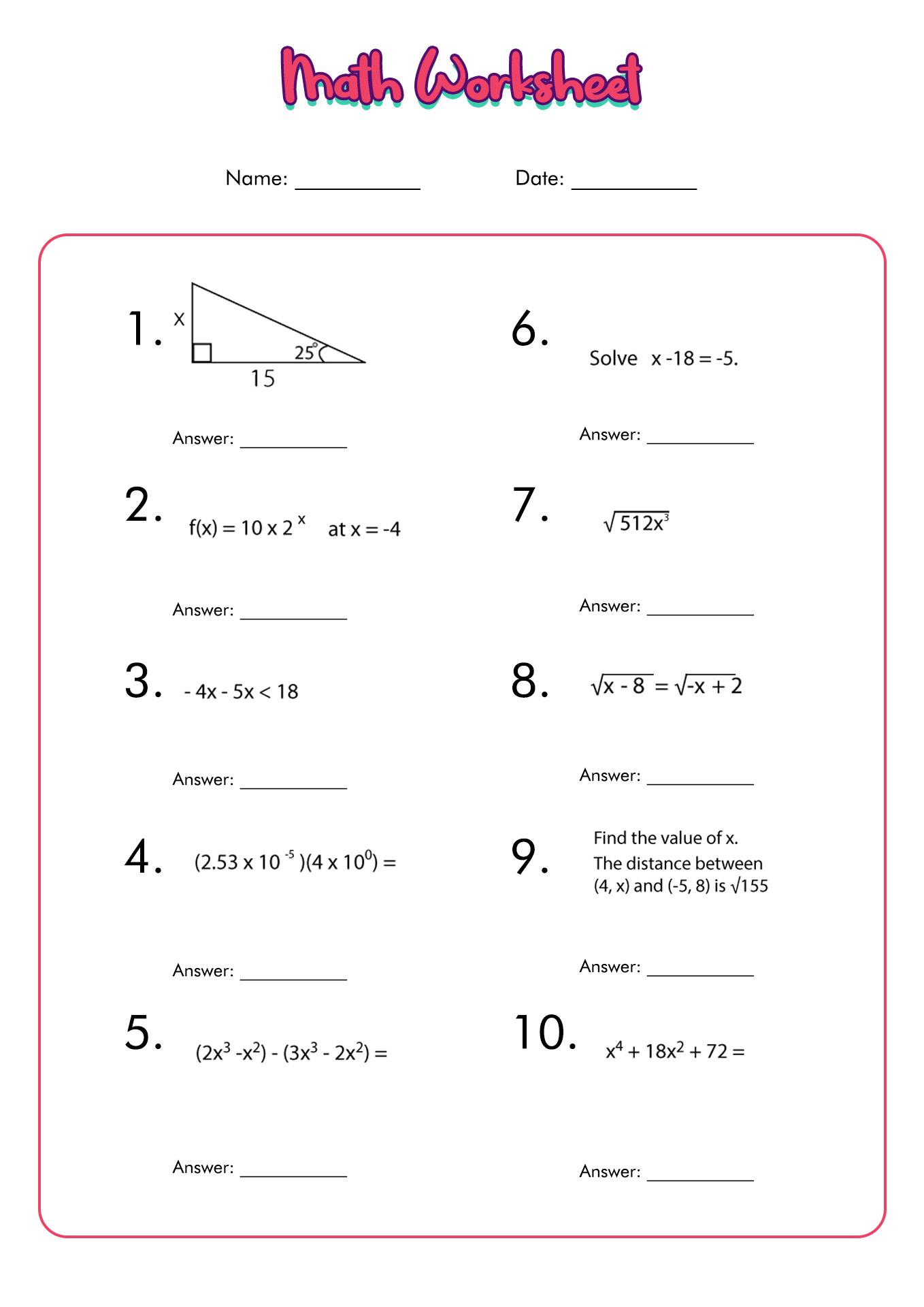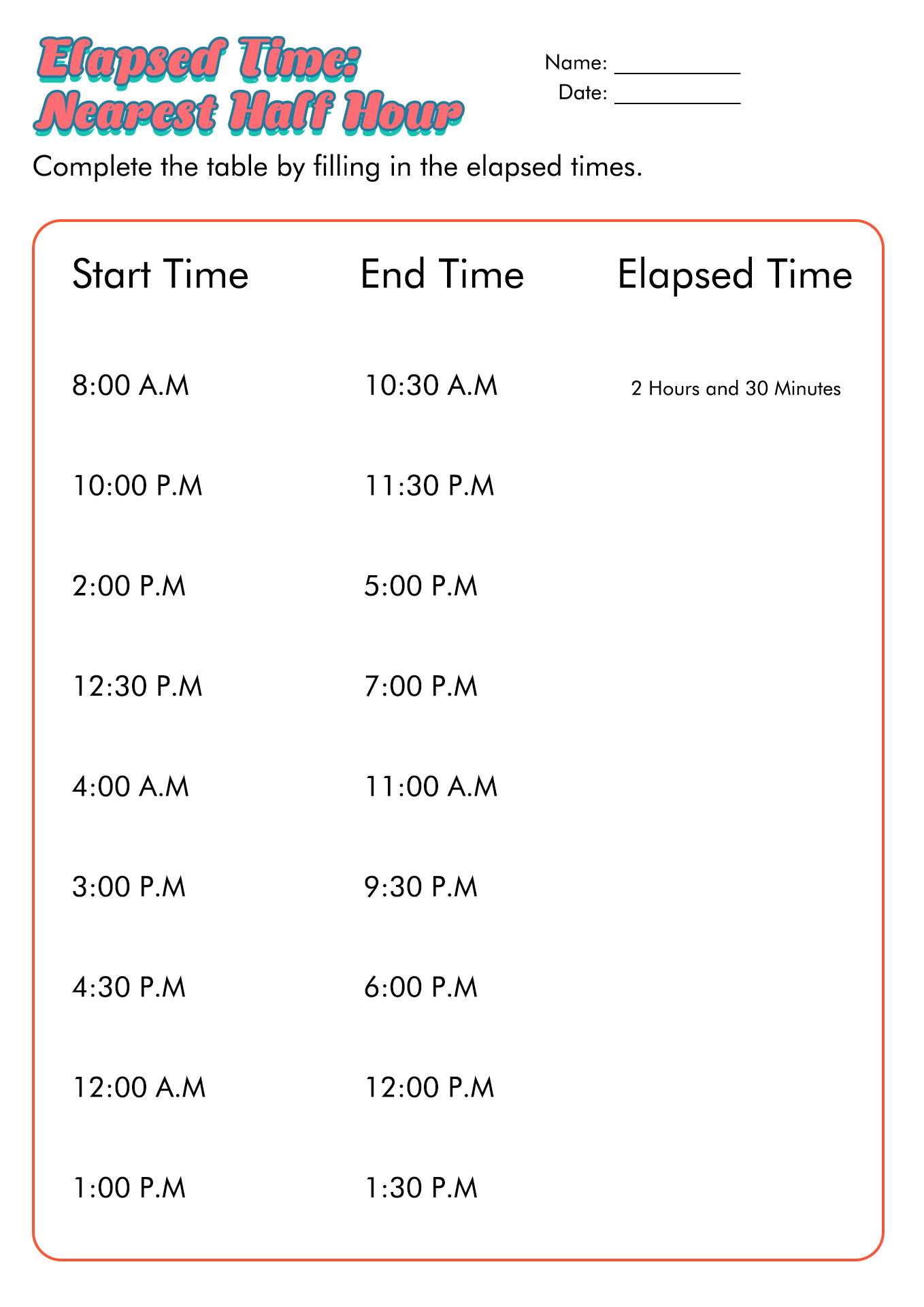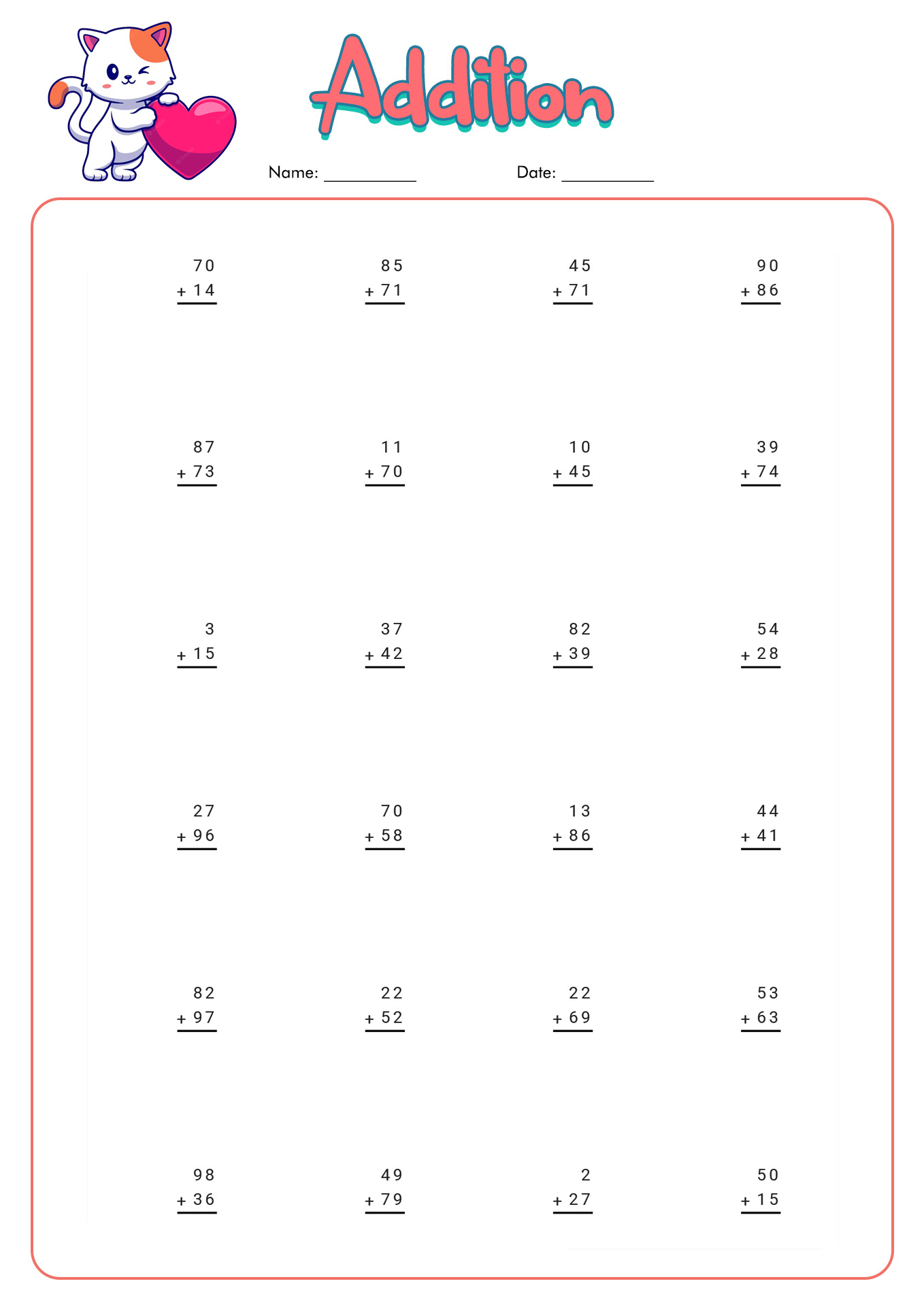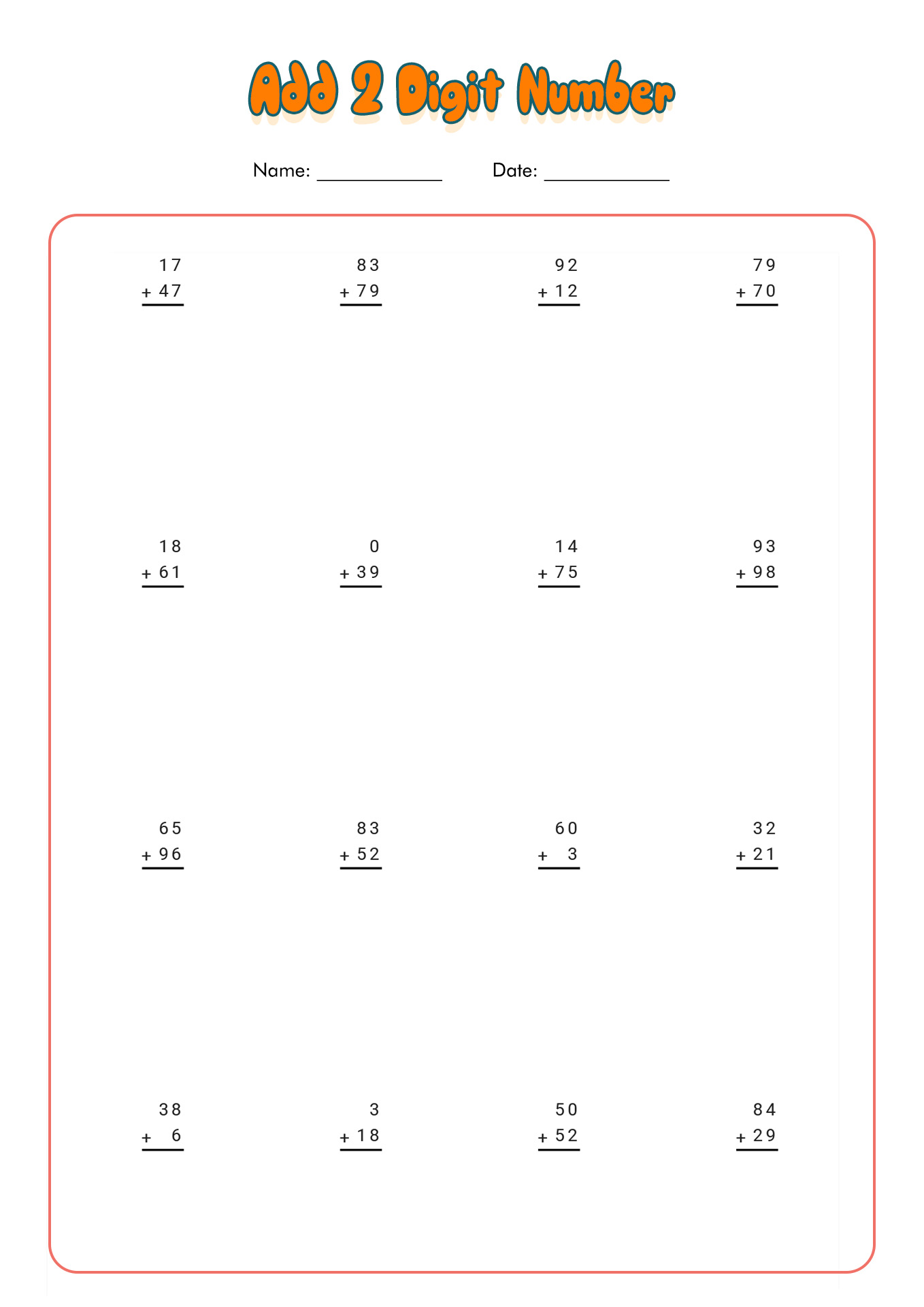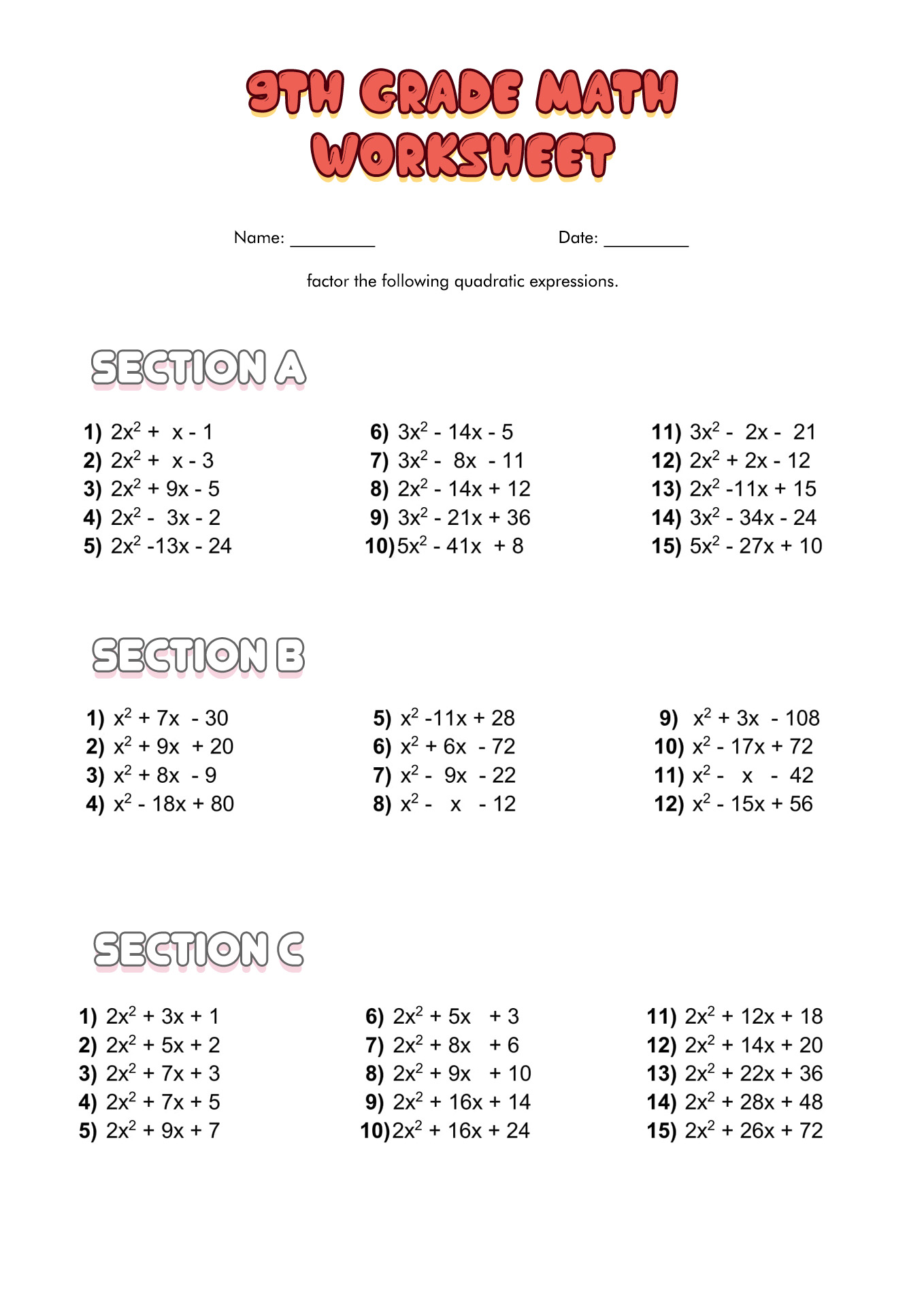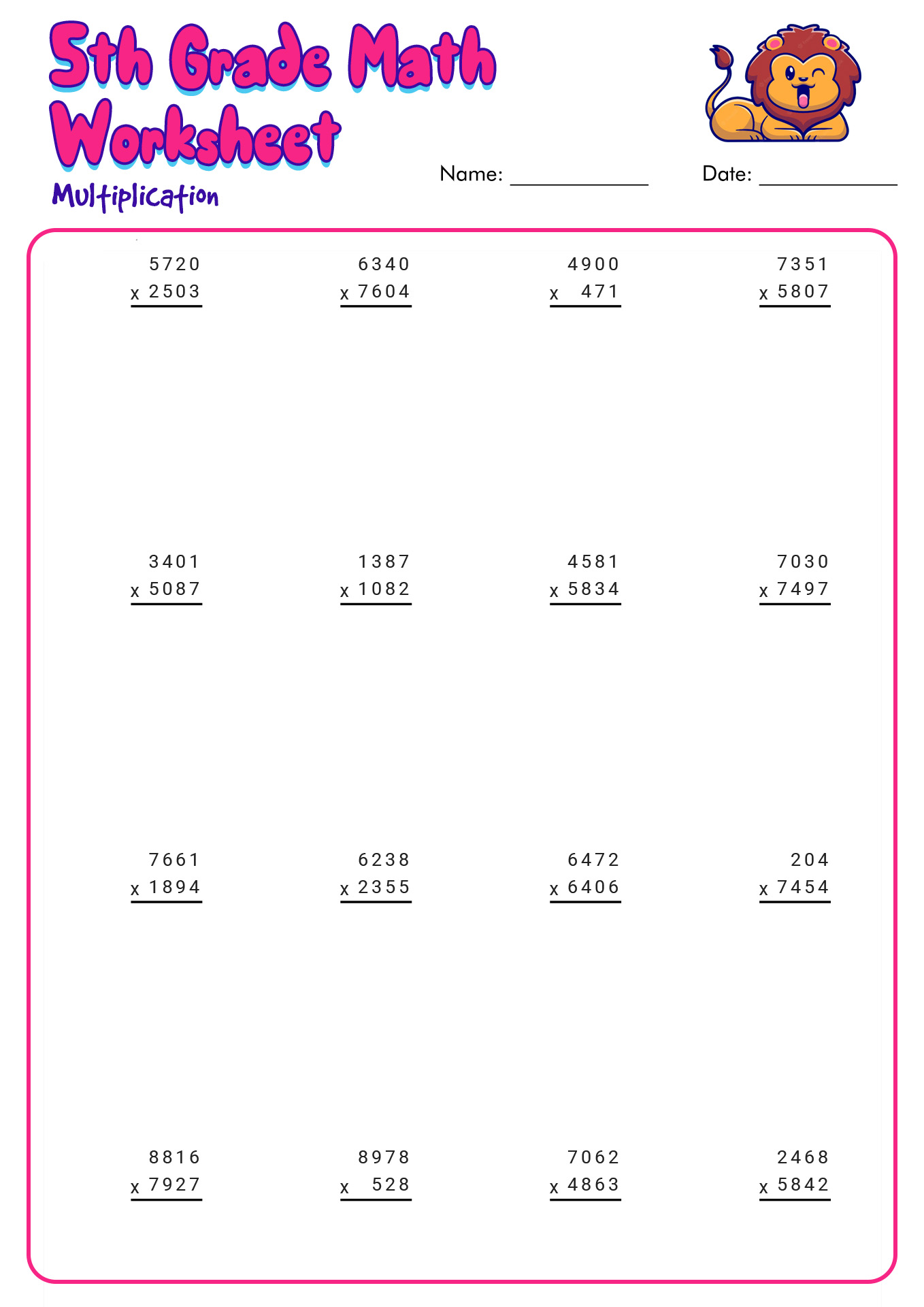Explore the numerical world through these Printable Math Worksheets!

Summary: Mathematics has an essential role in human everyday life. The components of mathematical skill include numerical knowledge, counting, computation, reasoning, math notation, and time and space reasoning. Mastering mathematics can help people in their education, jobs, and daily life. Hence, parents and teachers should teach their children and students the proper mathematic skills.

### What is Math Skill?

In these modern days, we all know the crucial role of numerical knowledge in human everyday life. It covers various aspects to help people in life. Hence, mastering mathematics is essential for people to ensure smooth activities. According to Neuropsychological Assessment in Schools by Daniel. C. Miller, the components of mathematical skill includes numerical knowledge, counting, computation, reasoning, math notation, and time and space reasoning. Mastering mathematics can help people in their education, jobs, and daily life. Even studying the essentials will help. It will serve as a bonus point to solve many problems. Here are some mathematical skills that can help people survive; some of them might be surprising to be included as math abilities: critical thinking, problem-solving, analytical thinking, quantitative reasoning, time management, building logical reasoning, abstract thinking, data analysis, research, numeracy abilities, forecasting, attention to details, mental arithmetic, and measuring profits.

### How Many Types of Math Skills Are There?

There are six dominant mathematical abilities that students should master to help them understand this numerical knowledge. Those six skills are arithmetic, algebra, geometry, calculus, statistics, and trigonometry. Arithmetic means the understanding of numerical values and elemental mathematical symbols. The students should be able to do arithmetic operations (addition, subtraction, division, and multiplication). Understanding terms such as sum, product, difference, and quotient is also part of the arithmetic skills. Next, algebra means comprehending the relation between variables and the rules to manipulate them. The coordinate plane is also the uniqueness of algebra. Third, Geometry is the part of mathematics where the students will learn about properties and their elements (distance, shape, size, points, lines, surface, dimension, and more). Another branch of mathematics is calculus. It is a part where the students will learn about spotting the properties of derivatives and integrals by methods based on the summation of infinitesimal distinctions. There are two types of calculus, differential and integral. Lastly, trigonometry is the mathematical side that deals with a particular relation of angles and length ratio.

### Why Should People Master Mathematics?

Many people agree that mathematics is one of the most difficult lessons to master. It might be because of the overwhelming exposure to numbers and other unfamiliar symbols. However, many also agree about the crucial role of mathematics in human daily life. Hence, parents and teachers should teach and introduce math to their children and students. Below are some benefits of mathematics:

• It will help the students to build the foundation for future complex studies, such as advanced science and engineering.
• Math will help humans to solve various daily problems (finance, estimating distance, cooking, baking, driving, and more).
• Mathematics can be a fun and exciting practice to help people relax their minds. For some people, solving a mathematical problem is like solving a puzzle.
• It will help to develop flexible, logical, and analytical thinking abilities.
• Learning mathematics means learning about cultural heritage since it is a historic cultural inheritance from our ancestors.
• Studying math can help people to enhance their problem-solving skills.
• It can improve creativity mind.
• Studying math will prepare students to learn more complex numerical concepts in their senior years.
• Learning mathematics will help students to open various doors to future jobs.

### How to Introduce Mathematic Skills to Children?

Teaching mathematics is as tough as learning it. Hence, parents and teachers should consider and ensure they give their children and students proper and appropriate math learning. Learning mathematical fundamentals is essential since it will be the background knowledge for the students in their future education. For early mathematic exposure, parents could trigger the children's numerical sense with various activities, such as shape learning by using blocks or paper, counting practice with toys and fingers, color sorting games with everyday objects, incorporating everyday activities with mathematics or using various visual media to help them study in a fun and exciting environment. Surround their days with mathematical situations in a fun way. This strategy will help them in the future, as they will not feel nervous about numbers.

### What are the Tips to Improve Math Skills?

Many students struggle to understand math, as it is a complicated concept of knowledge. Below are some tips and strategies to improve students' mathematical skills:

• Strengthen the mastery of basic math skills (addition, subtraction, division, multiplication, percentages, fractions and decimal, and spatial reasoning).
• Understand the concept before diving into repeated practices.
• Use mathematic games as practice tools and mediums.
• Find and solve the mathematics problem around yourself.
• Break down the steps of problem-solving to make it easier to understand.
• Enjoy the process of learning, and do not rush yourself.

The information, names, images and video detail mentioned are the property of their respective owners & source.

### Popular Categories

Have something to tell us about the gallery?

Submit